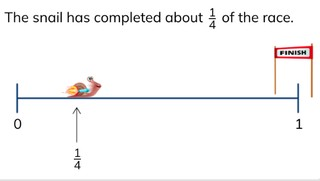Placing simple fractions on the number line

# Placing simple fractions on the number line

Students learn to place simple fractions on the number line.

No account needed.8,000 schools use Gynzy92,000 teachers use Gynzy1,600,000 students use Gynzy

## General

The students learn to place simple fractions on the number line.

## Standards

CCSS.Math.Content.3.NF.A.2

## Learning objective

Students will be able to place fractions on the number line.

## Introduction

Have the students read the fraction from the fraction bars. For the third problem the students fill in the fraction bar to match the fraction.

## Instruction

Explain that you first count how many parts the number line is divided up into in order to be able to place a fraction on the number line. Show that the number line is divided into four parts. The arrow is at the first division line. That is the first part of 4. The fraction that tells this is 1//4. Practice together with the students determining what fractions are indicated by the other arrows on the number line. After this you place the fractions on the other number lines together with the students. Next, check whether the students can place fractions on the number line on their own in the next three problems. Explain that if you have a number line without division lines, you must estimate the fraction that the arrow is indicating. You do this by dividing up the number line into equal parts until you see what division line the arrow is closest to. You can do this in your head or you can draw the division lines on the number line. Next you check whether the students can do this on their own through the following three exercises.

Check whether the students can place fractions on the number line by asking the following question:
- How do you figure out what fraction is on the number line without division lines?

## Quiz

The students test their understanding of placing simple fractions on the number line through ten exercises. In each exercise, they must determine the fraction that is indicated by the arrow on the number line. In some of the exercises, there are no division lines and the students have to estimate what fraction is shown by the arrow.

## Closing

Discuss with the students the importance of being able to place fractions on the number line. As a closing activity you can practice placing fractions on the number line together with the students. For the first number line you can drag the division lines and the arrow to the number line to make a fraction. For the second number line you can drag the arrow to the number line to estimate what the fraction is.

## Teaching tips

Students that have difficulty with placing fractions on the number line can first practice dividing a number line into a number of parts. Ask what fraction belongs with the number line. Next you have the students indicate what fraction is shown at each division line.

## Instruction materials

You may distribute sheets of paper with empty number lines on them.

### The online teaching platform for interactive whiteboards and displays in schools

• Save time building lessons

• Manage the classroom more efficiently

• Increase student engagement